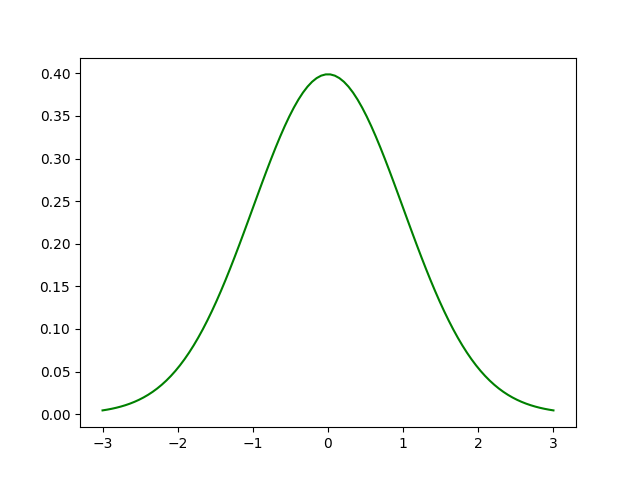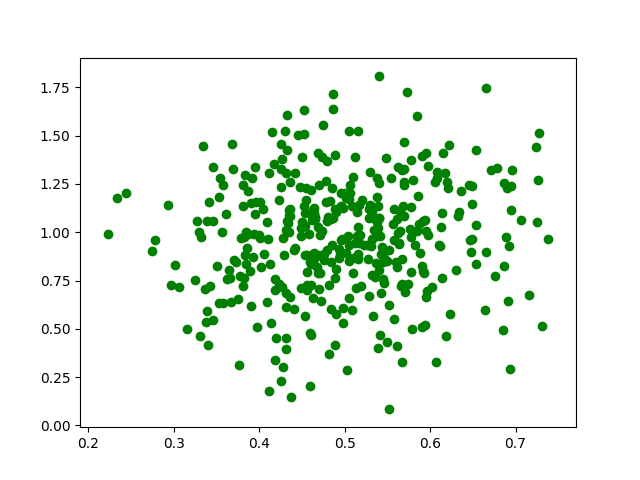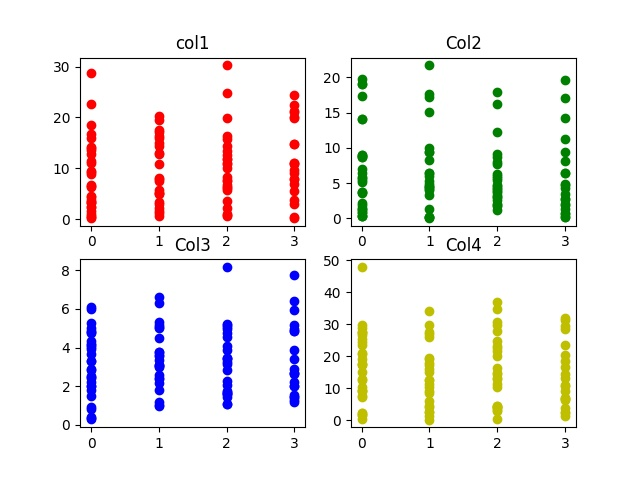# Generate test datasets for Machine learning

Whenever we think of Machine Learning, the first thing that comes to our mind is a dataset. While there are many datasets that you can find on websites such as Kaggle, sometimes it is useful to extract data on your own and generate your own dataset. Generating your own dataset gives you more control over the data and allows you to train your machine learning model.

In this article, we will generate random datasets using the Numpy library in Python.

Libraries needed:

```-> Numpy: sudo pip install numpy
-> Pandas: sudo pip install pandas
-> Matplotlib: sudo pip install matplotlib
```

## Normal distribution:

In probability theory, normal or Gaussian distribution is a very common continuous probability distribution that is symmetric about the mean, showing that data near the mean are more frequent in occurrence than data far from the mean. Normal distributions used in statistics and are often used to represent real-valued random variables.

The normal distribution is the most common type of distribution in statistical analyses. The standard normal distribution has two parameters: the mean and the standard deviation. The mean is the central tendency of the distribution. The standard deviation is a measure of variability. It defines the width of the normal distribution. The standard deviation determines how far away from the mean the values tend to fall. It represents the typical distance between the observations and the average. it fits many natural phenomena, For example, heights, blood pressure, measurement error, and IQ scores follow the normal distribution.

Graph of normal distribution:Example:

 `# importing libraries ` `import` `pandas as pd ` `import` `numpy as np ` `import` `matplotlib.pyplot as plt ` ` ` `# initialize the parameters for the normal ` `# distribution, namely mean and std. ` `# deviation ` ` ` `# defining the mean ` `mu ``=` `0.5` `# defining the standard deviation  ` `sigma ``=` `0.1` ` ` `# The random module uses the seed value as a base  ` `# to generate a random number. If seed value is not  ` `# present, it takes the system’s current time. ` `np.random.seed(``0``) ` ` ` `# define the x co-ordinates ` `X ``=` `np.random.normal(mu, sigma, (``395``, ``1``)) ` ` ` `# define the y co-ordinates ` `Y ``=` `np.random.normal(mu ``*` `2``, sigma ``*` `3``, (``395``, ``1``)) ` ` ` `# plot a graph ` `plt.scatter(X, Y, color ``=` `'g'``) ` `plt.show() `

Output :Let us look at a better example.

We will generate a dataset with 4 columns. Each column in the dataset represents a feature. The 5th column of the dataset is the output label. It varies between 0-3. This dataset can be used for training a classifier such as a logistic regression classifier, neural network classifier, Support vector machines, etc.

 `# importing libraries ` `import` `numpy as np ` `import` `pandas as pd ` `import` `math ` `import` `random ` `import` `matplotlib.pyplot as plt  ` ` ` `# defining the columns using normal distribution ` ` ` `# column 1 ` `point1 ``=` `abs``(np.random.normal(``1``, ``12``, ``100``)) ` `# column 2 ` `point2 ``=` `abs``(np.random.normal(``2``, ``8``, ``100``)) ` `# column 3 ` `point3 ``=` `abs``(np.random.normal(``3``, ``2``, ``100``)) ` `# column 4 ` `point4 ``=` `abs``(np.random.normal(``10``, ``15``, ``100``)) ` ` ` `# x contains the features of our dataset ` `# the points are concatenated horizontally ` `# using numpy to form a feature vector. ` `x ``=` `np.c_[point1, point2, point3, point4] ` ` ` `# the output labels vary from 0-3 ` `y ``=` `[``int``(np.random.randint(``0``, ``4``)) ``for` `i ``in` `range``(``100``)] ` ` ` `# defining a pandas data frame to save ` `# the data for later use ` `data ``=` `pd.DataFrame() ` ` ` `# defining the columns of the dataset ` `data[``'col1'``] ``=` `point1 ` `data[``'col2'``] ``=` `point2 ` `data[``'col3'``] ``=` `point3 ` `data[``'col4'``] ``=` `point4 ` `     ` `# plotting the various features (x) ` `# against the labels (y). ` `plt.subplot(``2``, ``2``, ``1``) ` `plt.title(``'col1'``) ` `plt.scatter(y, point1, color ``=``'r'``, label ``=``'col1'``) ` `     ` `plt.subplot(``2``, ``2``, ``2``) ` `plt.title(``'Col2'``) ` `plt.scatter(y, point2, color ``=` `'g'``, label ``=``'col2'``) ` `     ` `plt.subplot(``2``, ``2``, ``3``) ` `plt.title(``'Col3'``) ` `plt.scatter(y, point3, color ``=``'b'``, label ``=``'col3'``) ` `     ` `plt.subplot(``2``, ``2``, ``4``) ` `plt.title(``'Col4'``) ` `plt.scatter(y, point4, color ``=``'y'``, label ``=``'col4'``) ` `     ` `# saving the graph ` `plt.savefig(``'data_visualization.jpg'``)         ` ` ` `# displaying the graph ` `plt.show() `

Output :# Special 95% discount

## 2000+ Applied Machine Learning & Data Science Recipes

### Portfolio Projects for Aspiring Data Scientists: Tabular Text & Image Data Analytics as well as Time Series Forecasting in Python & R## Two Machine Learning Fields

There are two sides to machine learning:

• Practical Machine Learning:This is about querying databases, cleaning data, writing scripts to transform data and gluing algorithm and libraries together and writing custom code to squeeze reliable answers from data to satisfy difficult and ill defined questions. It’s the mess of reality.
• Theoretical Machine Learning: This is about math and abstraction and idealized scenarios and limits and beauty and informing what is possible. It is a whole lot neater and cleaner and removed from the mess of reality.

Data Science Resources: Data Science Recipes and Applied Machine Learning Recipes

Introduction to Applied Machine Learning & Data Science for Beginners, Business Analysts, Students, Researchers and Freelancers with Python & R Codes @ Western Australian Center for Applied Machine Learning & Data Science (WACAMLDS) !!!

Latest end-to-end Learn by Coding Recipes in Project-Based Learning:

Applied Statistics with R for Beginners and Business Professionals

Data Science and Machine Learning Projects in Python: Tabular Data Analytics

Data Science and Machine Learning Projects in R: Tabular Data Analytics

Python Machine Learning & Data Science Recipes: Learn by Coding

R Machine Learning & Data Science Recipes: Learn by Coding

Comparing Different Machine Learning Algorithms in Python for Classification (FREE)

`Disclaimer: The information and code presented within this recipe/tutorial is only for educational and coaching purposes for beginners and developers. Anyone can practice and apply the recipe/tutorial presented here, but the reader is taking full responsibility for his/her actions. The author (content curator) of this recipe (code / program) has made every effort to ensure the accuracy of the information was correct at time of publication. The author (content curator) does not assume and hereby disclaims any liability to any party for any loss, damage, or disruption caused by errors or omissions, whether such errors or omissions result from accident, negligence, or any other cause. The information presented here could also be found in public knowledge domains.  `